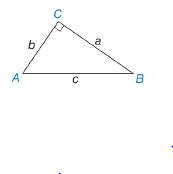Chapter 3.2, Problem 24E### Elementary Geometry for College St...

6th Edition
Daniel C. Alexander + 1 other
ISBN: 9781285195698

#### Solutions

Chapter
Section### Elementary Geometry for College St...

6th Edition
Daniel C. Alexander + 1 other
ISBN: 9781285195698
Textbook Problem
1 views

# In Exercise 21 to 26, Δ A B C is a right triangle. Use the given information to find the length of the third side of the triangle. b = 6 and c = 10To determine

To find:

The length of the third side of the triangle.

Explanation

Given:

The following figure shows the given diagram.

Given that,

b=6 and c=10

Approach:

The given triangle is right angle triangle and C is right angle then side AB¯ will be hypotenuse.

The Pythagoras theorem is given by,

c2=a2+b2

Calculation:

Substitute 6 for b, 10 for c in the above mentioned equation to obtain the value of a.

### Still sussing out bartleby?

Check out a sample textbook solution.

See a sample solution

#### The Solution to Your Study Problems

Bartleby provides explanations to thousands of textbook problems written by our experts, many with advanced degrees!

Get Started